# RRB JE Quant quiz for Phase I and other railways exams 30/01/2019

Quant quiz for RRB JE (Phase I), RRB NTPC, SSC various exams and other competitive exams.

Direction (Q1 – Q4): Study the following line graph showing the expenditures of six persons to answer the questions that follow.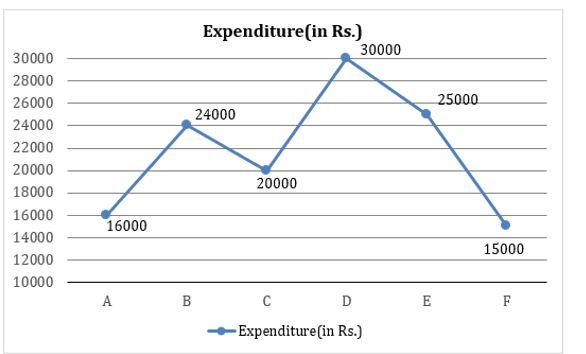NOTE: Income = Expenditure (Bill + House Rent + Clothing + Food) + Savings (25% of income)

Q1. If the expenditure of E on bill is equal to the expenditure of B on bill(which is 10% of his income). If income of B is decreased by 12.5% then expenditure on bills for person E is changed by what % ?

A) 6000 Rs.

B) 8000 Rs.

C) 10000 Rs.

D) 12000 Rs.

Q2. If D & F spend 20% & 33 1/3 % of their monthly expenditure on house rent, then expenditure of B on Education (which is 10% of his total income) is what percent of expenditure of D & F together on house rent?

A) 27 1/11%

B) 25 1/11%

C) 29 1/11%

D) None of these

Q3. Saving of C is what % more or less than saving of B?

A) 16 2/3%

B) 12.5%

C) 11 1/9%

D) 14 2/7%

Q4. If the expenditure of E on bill is equal to the expenditure of B on bill(which is 10% of his income). If income of B is decreased by 12.5%then expenditure on bills for person E is changed by what % ?

A) 7.5%

B) 12.5%

C) 5%

D) 15%

Q5. The lines x, y and z are parallel. If AB = 4 cm BC = 6cm, AD = 12 cm and FG =DE then find EF .A) 25 cm

B) 21 cm

C) 27 cm

D) 22 cm

Q6. The average weight of 3 men A,B and C is 85 kg another man D joins the group and the average now become 81 kg. If another man E whose weight is 4 kg more than that of D, replaces A then the average weight of B, C, D and E becomes 80 kg. What is the weight of A?

A) 71 kg

B) 70 kg

C) 75 kg

D) 77 kg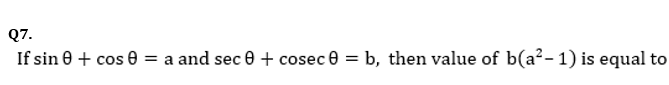A) 0

B) – 2a

C) a

D) 2a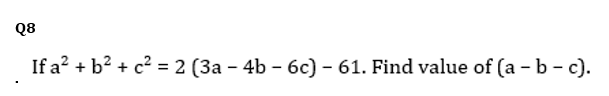A) 11

B) 17

C) 13

D) 19

Q9. Work done by (x + 4) men in (x + 5) days is equal to the work done by (x – 5) men in (x + 20) days. The value of x is

A) 20

B) 12

C) 16

D)  15A) 3

B) 6

C) 2

D)  4

# Solutions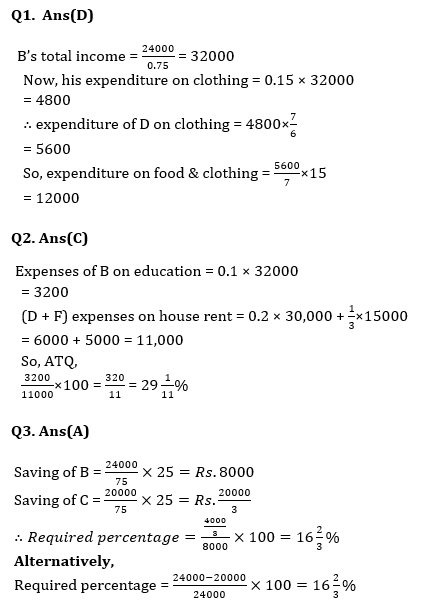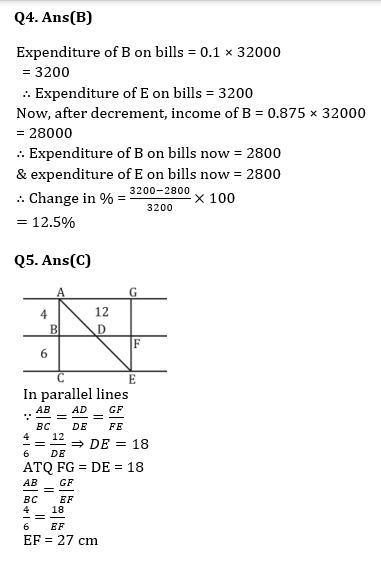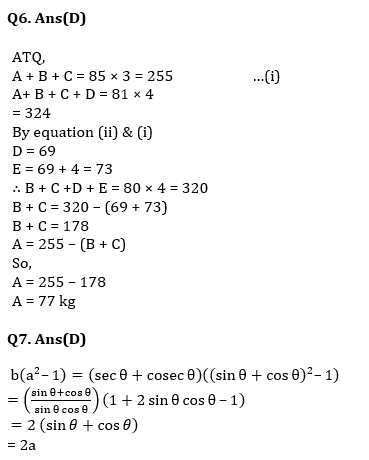## WhatsApp Group Join here

Mail us at : ambitiousbaba1@gmail.com